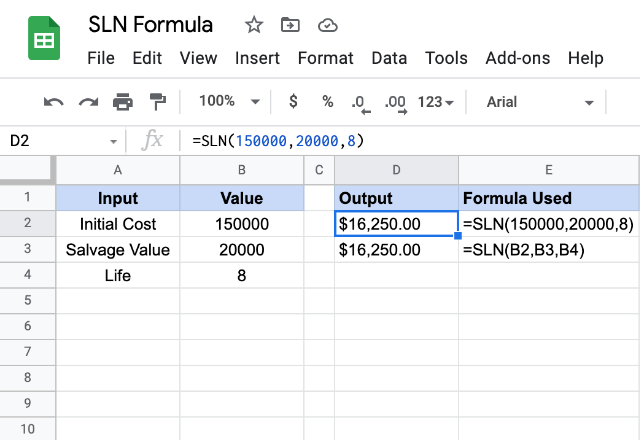# How to use the SLN formula in Google SheetsThere are usually two kinds of assets – appreciating and depreciating assets. While investments such as stocks, bonds etc. fall into the former category, the purchases like a house and car belong to the latter category. Knowing how an asset depreciates plays a vital role in buying decisions. The SLN formula in Google Sheets employs a straight line method of calculating the depreciation an asset undergoes over a period of time. It considers three different input values as explained in below.

### Syntax

SLN(cost, salvage, life)

• cost – is the initial acquisition price of the asset.
• salvage – is the value of the asset at the end of depreciation. It can be thought of as the resale value after a certain depreciating period.
• life – is the number of periods the asset keeps depreciating for.

### Usage: SLN formula in Google Sheets

The syntax of this formula is relatively simple when compared to other myriad financial formulas that Google Sheets is packed with. Nevertheless, we will try and practice this on the Google Sheets application to understand it better. Please have a look at the following image.As with the other formulas in Google Sheets, we can either use direct numeric values for the input parameters of the SLN formula. We demonstrated this in the first example. Or we could also use the cell references as is the case with the second example.

If we try and analyze the working of this formula, it is probably very straightforward as to how the formula works. As indicated in the first paragraph, this formula uses straight-line method to calculate the depreciation amount. For ease of our understanding, if we were to assign a variable letter to each input as below:

• D = depreciation amount per period
• C = Initial acquisition cost
• S = salvage value of the asset at the end of its life
• L = number of periods

The SLN formula should be evaluating the output values using the following equation:

D = (C – S) / L

### SLN formula

And there you go! Use the SLN formula in Google Sheets to calculate the depreciation an asset undergoes over a period of time.

If you’d like to learn more about the various formulas of Google Sheets, why not take a look at our blog posts on the DISC formula in Google Sheets

Alternatively, check out related blog posts below!